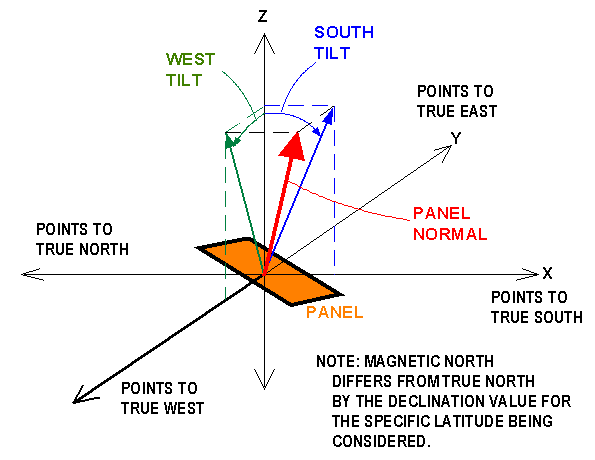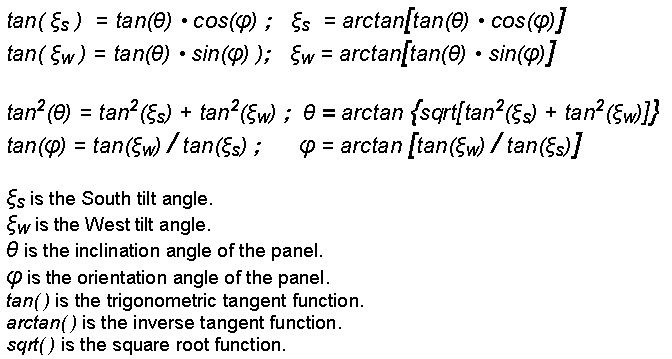Definition of South Tilt and West Tilt Angles
 There are a number of coordinate systems for specifing the orientation of a solar panel -- more specifically, the orientation of the normal vector of the panel.  The normal vector of a panel is the striaght line which is perpendicular to the surface of the panel.  See the red arrow in the below figure, which is perpendicular to the orange panel.    For all the coordinate systems that we discuss here, wer will use an underlying XYZ-Cartesian reference system where the X-axis points to true South, the Y-axis points to true East, and the Z-axis points directly up.  The XYZ-Cartesian reference system will easily enable us to derive conversion formulas between the various coordinate systems. .  The computer program uses the system which specifies the orientation of the panel's normal vector with the two angles: South tilt and West tilt, as best defined in the following figure:Technically, the South Tilt is the angle between the Z-axis and the projection vector of the Panel Normal in the X-Z plane, as shown by the blue lines above. Similarly, the West Tilt is the angle between the Z-axis and the projection vector of the Panel Normal in the Y-Z plane, as shown by the green lines above.       While this coordinate system is great for computing sun exposure over the year, it is not easy to work with when planning and installing a solar panel array.  A better coordinate system for planning and installing is shown below, with the panel inclination angle and teh panel orientation angle:The inclination angle (theta) is the tilt angle of the panel normal with respect to the Z-axis, which is equal to the tilt angle of the panel surface with respect to the ground.  For example, if the panel is mounted on a roof that rises 3 feet for every 12 feet of horzontal run, then the inclination angle is arctan(3/12) = 14 degrees, where arctan is the inverse tangent function.  The inclination angle is always positive.    To define the orientation angle (phi), we draw an imaginary straight line that is parallel to the bottom edge of the panel.  The orientation angle is the angle between this imaginary line and the Y-axis, as shown above for a positive value of the orientation angle.  A more precise way of defining the orientation angle is to draw a vector which is perpendicular to the bottom edge of the pannel AND which lies only in the X-Y plane.  For an orientation angle of zero, this vector points directly to true South, and the bottom edge of the panel is parallel to the Y axis (East-West axis).    The following formulas provide a conversion between the two systems:As an example, a panel with a 3-12 pitch would have an inclination angle of tan(3/12)=14 degrees and an orientation angle of 45 degrees would have a South tilt of 11 degrees and a West tilt of 11 degrees.